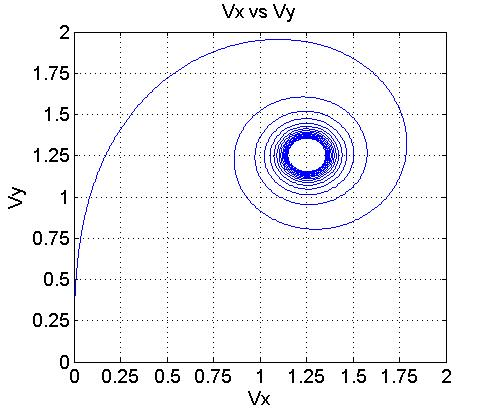# Q & A: Pushing on a bar at one end

Q:
This is a thought experiment: Assume there is a bar of some rigid material of length L [meters], and mass per unit length m [kg/m/. The bar is free floating in outer space, so has neglegible gravity acting on it. One end of the bar has a constant force F [Newtons] applied at a right angle to the bars length axis. The force is always applied in the same orientation with respect to the bar.My question is what path will the bar follow. Will it simply rotate about it's centre of mass, or will it describe a spiral motion?
- Simon Bagley (age 53)
Stockport, Cheshire, UK
A:

Hello Simon,

This is a cute little problem.  I gave up trying to solve the thing analytically so decided to code it up using MATLAB.   Here is a plot showing the evolution of Vx vs Vy.   Asymptotically, the bar bar spins faster and faster and moves off at a 450 angle with a more or less constant velocity.LeeH

One way to get a qualitative feel is to think first of the rotations. These are simple because there's a constant torque around the center of mass. So the rotations keep speeding up, with angular speed growing linearly in time. That's why, after a while, the velocity becomes nearly constant. The direction of the applied force is spinning around so quickly that it nearly averages zero. /Mike W.

(published on 12/15/2014)